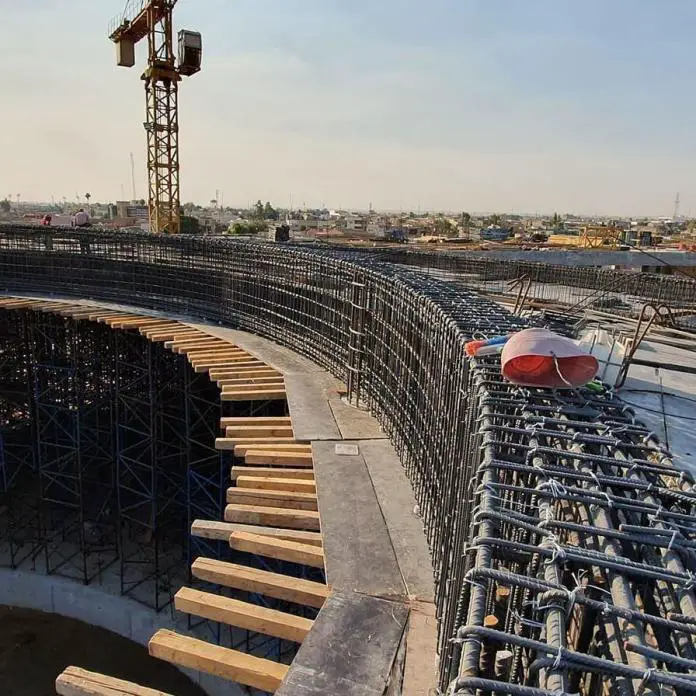# Minimum Area of Reinforcement Required for Reinforced Concrete BeamsIn reinforced concrete beams, the minimum area of longitudinal reinforcement required is given by;

As,min = 0.26 (fctm / fykbt d   ≥ 0.0013 bt d —- (1)

where:
fctm is the mean value of axial tensile strength of concrete at 28 days, see Table 3.1 of EN 1992-1-1:2004
fyk is the characteristic yield stress of reinforcement steel
bt is the mean width of the tension zone; for a T-beam with the flange in compression, only the width of the web is taken into account in calculating the value of bt
d is the effective depth of concrete cross-section.

Any section containing less than As,min as given in equation (1) should be treated as an unreinforced section.

The minimum area of reinforcement for different classes of concrete strengths assuming fyk = 500 MPa is given in the Table below;

For a T-beam with the flange in tension (such as upstand beams or ground beams), the minimum area of reinforcement is given by equation (2);

As,min = 0.26 (fctm / fykAc,t —– (2)

Where Ac,t is the area of the tension zone above the neutral axis of the reinforcement.

BS 8110-1:1997 made express provisions for flanged beams for different stress states of the web or the flange. The requirements are given in the table below;

Maximum area of longitudinal reinforcement
The maximum area of tension and compression reinforcement in reinforced concrete beams is;

As,max = 0.04Ac —- (3)

Where;
Ac is the area of the cross-section

The minimum area of shear reinforcement in beams should be calculated from;

Asw/sbw ≥ ρw,min —– (4)

Where;
ρw,min = (0.08√fck)/fyk
Asw = Area of shear reinforcement
s = spacing of shear reinforcement
bw = width of the web

Solved Example
For the concrete section shown below, find the minimum area of longitudinal reinforcement required for;
(a) When the web is in tension
(b) When the flange is in tension
fck = 25 MPa, fyk = 500 MPa

(a) When the web is in tension
Using Eurocode;
As,min = 0.26 (fctm / fykbt d
As,min = 0.26 x (2.6/500) x 250 x 695 = 235 mm2 > 0.0013btd (0.0013 x 250 x 695) = 226 mm2

Using Table 3.25, BS 8110-1:1997;
bw/b = 250/1500 = 0.167 < 0.4
As,min = 0.18bwh/100 = (0.18 x 250 x 750)/100 = 338 mm2

(b) When the flange is in tension
Using Eurocode;
As,min = 0.26 (fctm / fykAc,t
Ac,t = (550 x 250) + (1500 x 145) = 355000 mm2
As,min = 0.26 x (2.6/500) x 355000 = 480 mm2

Using Table 3.25, BS 8110-1:1997;
As,min = 0.26bwh/100 = (0.26 x 250 x 750)/100 = 488 mm2

#### 1 COMMENT

1.Nicolas MARIE

Hi. Would you please discuss how is the following down stand beam considered in design calculation: The web is cast first. The flange is cast during the casting of the slab. Say for example the beam is 150×425 with the 425mm. including 125mm. for the thickness of the slab.